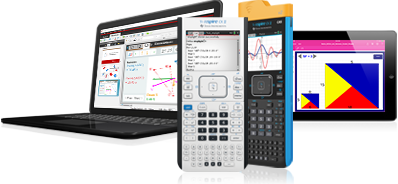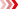Precalculus
Find activities that support your lesson plans

Challenge students to use and think about how technology can be used to model, analyze and explain math with standards-aligned activities in eight precalculus topics.

Activities can be used as is or edited to support specific objectives, align with popular text books and include technology tips to help you focus the learning and address student misconceptions.# Featured Activities

## Exploring Vertical Asymptotes

This lesson involves observing how changing the values in a rational function affects the continuity of the graph of the function. Students will explain how the values in a rational function determine the vertical asymptotes.## Crossing the Asymptote

This lesson involves the graph of a rational function of the form r(x) = p(x) / q(x). Students will manipulate graphs of rational functions and their asymptotes to determine whether they intersect.## Polar Coordinates

This lesson involves a brief introduction to the polar coordinate system. Students will determine the location (quadrant) of various points given in polar form and recognize cases in which a point lies on an axis. Students will convert points between polar and rectangular form.